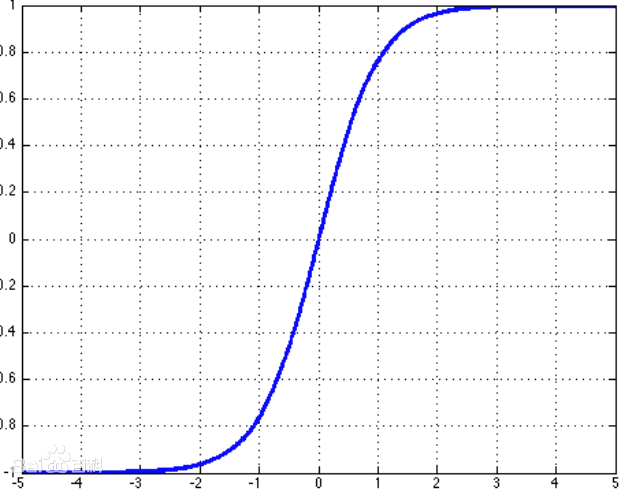# 权值初始化 - Xavier和MSRA方法

1. 初始化权值参数
3. 重复下面的迭代过程：
1. 输入的正向传播
2. 计算loss function 的值
3. 反向传播，计算loss function 相对于权值参数的梯度值
4. 根据选择的梯度下降算法，使用梯度值更新每个权值参数

### Xavier初始化

Xavier初始化，由Xavier Glorot 在2010年的论文 Understanding the difficulty of training deep feedforward neural networks 提出。

1. 每一层神经元激活值的均值要保持为0
2. 每一层激活的方差应该保持不变。

\begin{aligned} W^{[l]} & \sim \mathcal{N}\left(\mu=0, \sigma^{2}=\frac{1}{n^{[l-1]}}\right) \\ b^{[l]} &=0 \end{aligned}

1. 权值矩阵$w$是独立同分布的，且其均值为0
2. 每一层的输入$a$是独立同分布的，且均值也为0
3. $w$$a$是相互独立的

$L$层的权值矩阵为$W$，偏置为$b$,其输入为$a$

$z^l = w^la^{l-1} + b^l$

$Var(z^l) = Var(\sum_{i=0}^nw_{i}^la_i^l) = \sum_{i=0}^n Var(w_{i}^la_i^{l-1})$

$Var(w_ix_i) = E^2(w_i)Var(w_i) + E^2(x_i)Var(x_i) + Var(w_i)Var(x_i)$

$Var(z^l) = \sum_{i=0}^nVar(w_i^l)Var(x_i^{l-1})$

$Var(z^l) = n^{l-1}Var(w^l)Var(a^{l-1}),(n-1)为上一层神经元的个数$

$f$为激活函数，则有

$a^l = f(z^l)$

Xavier假设的激活函数为$tanh$，其函数曲线为$a^l = tanh(z^l)$

$Var(a^l) = Var(z^l) = n^{l-1}Var(w^l)Var(a^{l-1})$

$Var(w^l) = \frac{1}{n^{l-1}}$

$\forall i, \operatorname{Var}\left(W^{l+1}\right)=\frac{2}{n_{l}+n_{l+1}}$

• 各个层激活值的方差保持不变（正向传播）
• 各个层的梯度值的方差保持不变（反向传播）

1. 权值矩阵$w$是独立同分布的，且其均值为0
2. 每一层的输入$a$是独立同分布的，且均值也为0
3. $w$$a$是相互独立的

Xavier的初始化有个假设条件，激活函数关于0对称，且主要针对于全连接神经网络。适用于tanh和softsign。

#### 均匀分布

$\forall i, \operatorname{Var}\left(W^{l+1}\right)=\frac{2}{n_{l}+n_{l+1}}$

$[a,b] $间的均匀分布的方差为$ var = \frac{(b-a)^2}{12}$,设$F_{in}$为输入的神经元个数，$F_{out}$为输出的神经元个数

$limit = \sqrt{\frac{6}{F_{in} + F_{out}}}$

$W \sim U[-limit,limit] \rightarrow W \sim U\left[-\sqrt{\frac{6}{F_{in} + F_{out}}}, + \sqrt{\frac{6}{F_{in} + F_{out}}}\right]$

#### 正态分布

$W \sim N(0.0,\sqrt{\frac{2}{F_{in} + F_{out}}})$

### He初始化（MSRA）

$w \sim G\left[0, \sqrt{\frac{2}{F_{in }}}\right]$

$\mathbf{y}_{l}=\mathbf{W}_{l} \mathbf{x}_{l}+\mathbf{b}_{l}$

$\operatorname{Var}\left[y_{l}\right]=n_{l} \operatorname{Var}\left[w_{l} x_{l}\right]$

$w_l$的均值为0，即$E(w_l) = 0$,则有：

\begin{align*} \operatorname{Var}(y_l) & = n_{l}(E(W_l^2) \cdot E(x_l^2) - E^2(w_l) \cdot E^2(x_l)) \\ &= n_{l}(E(W_l^2) \cdot E(x_l^2) - 0 \cdot E^2(x_l)) \\ & = n_{l}(E(W_l^2) \cdot E(x_l^2) - 0 \cdot E(x_l^2)) \\ & = n_{l}(E(W_l^2) \cdot E(x_l^2) - E^2(w_l) \cdot E(x_l^2)) \\ & = n_{l}(E(W_l^2) - E^2(w_l)) \cdot E(x_l^2) \\ & = n_{l} \operatorname{Var}(w_l) \cdot E(x_l^2) \end{align*}

$\operatorname{Var}(y_l) = n_{l} \operatorname{Var}(w_l) \cdot E(x_l^2)$

\begin{align*} \operatorname{E}(y_l) &= \operatorname{E}(w_lx_l) \\ &= \operatorname{E}(x_l) \cdot \operatorname{E}(w_l) \\ &= 0 \end{align*}

$x_l = max(0,y_{l-1})$

\begin{align*} \operatorname{E}(x_l^2) &=\frac{1}{2} \operatorname{E}(y_{l-1}^2） \\ &= \frac{1}{2}({E}(y_{l-1}^2) - E(y_{l-1})),(由于E(y_{l-1}) = 0）\\ & = \frac{1}{2}\operatorname{Var}(y_{l-1}) \end{align*}

$\operatorname{Var}\left[y_{l}\right]=\frac{1}{2} n_{l} \operatorname{Var}\left[w_{l}\right] \operatorname{Var}\left[y_{l-1}\right]$

$\operatorname{Var}\left[y_{L}\right]=\operatorname{Var}\left[y_{1}\right]\left(\prod_{l=2}^{L} \frac{1}{2} n_{l} \operatorname{Var}\left[w_{l}\right]\right)$

$\frac{1}{2} n_{l} \operatorname{Var}\left[w_{l}\right]=1, \quad \forall l$

$\sqrt{2 / n_{l}}$

$W \sim N(0.0,\sqrt{2 / n_{l}})$

$W \sim U[-\sqrt{6 / n_{l}},\sqrt{6 / n_{l}}]$

### 总结及使用的概率公式

• 各个层激活值的方差保持不变（正向传播）
• 各个层的梯度值的方差保持不变（反向传播）

$D(x)=E\left(x^{2}\right)-E^{2}(x)$

$D(x y)=E\left(x^{2} y^{2}\right)-E^{2}(x y)=E\left(x^{2}\right) E\left(y^{2}\right)-E^{2}(x) E^{2}(y)$

$D(xy) = D(y)E(x^2)$

$E(xy) = E(x)E(y)$

posted @ 2019-06-13 00:32  Brook_icv  阅读(4470)  评论(1编辑  收藏